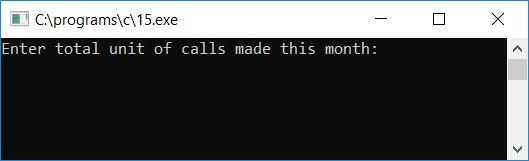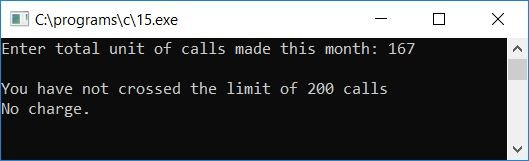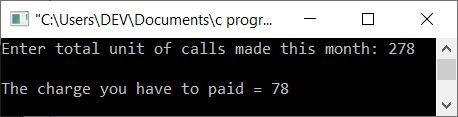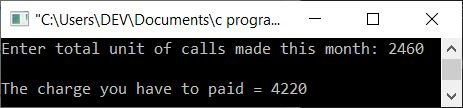# C Program to Calculate Telephone Call Bills

In this tutorial, we will learn how to create a program in C that will ask the user to enter the total number of telephone calls made in a particular month. Here, "number of telephone calls" means "number of minutes." After receiving the total number of phone calls made in a month, the program will calculate and print the payable amount (for that month) as per the given call rate:

• The first 200 phone calls are free every month.
• The next 300 calls are charged at 1 cents per minute.
• Rest calls (after 500 calls or minutes) are charged at a rate of 2 cents per minute.

Let's take a look at the program.

```#include<stdio.h>
#include<conio.h>
int main()
{
int noOfCall, tempNoOfCall, charge;
printf("Enter total unit of calls made this month: ");
scanf("%d", &noOfCall);
printf("\n");
if(noOfCall<=200)
{
printf("You have not crossed the limit of 200 calls");
printf("\nNo charge.");
}
else
{
if(noOfCall<=500)
{
tempNoOfCall = noOfCall - 200;
charge = tempNoOfCall * 1;
printf("The charge you have to paid = %d", charge);
}
else
{
tempNoOfCall = noOfCall - 500;
charge = tempNoOfCall * 2;
charge = charge + 300;
printf("The charge you have to paid = %d", charge);
}
}
getch();
return 0;
}```

Because the above program was written in the Code::Blocks IDE, here is the similar output you will see on your output screen after a successful build and run; this is the first snapshot of the sample run:The user must enter the number of calls (total number of units or minutes) made in the previous month here. Assume, for example, that the telephone user made 167 phone calls in the previous month. Therefore, the user has to supply the input as 167 and then press the ENTER key to see the charge that has to be paid for the previous month, as shown in the second snapshot of the sample run given here:Because the first 200 calls or minutes of talk are free, and the user has only made 167 minutes of calls, there is no charge for the previous month. Here is another sample run, but this time the user has crossed the limit of 200 free calls:Here is another sample run. This time, let's suppose that the user has made a total of 2460 minutes of calls in the previous month. Therefore, the charge is shown in the sample run given below:Here are some of the main steps used in the above program:

• Receive the total number of calls or minutes made in the previous month by the telephone user.
• Now check whether the input is less than or equal to 200 or not.
• If it is, then print a message saying you have not crossed the limit of the first 200 calls, therefore the telephone user has not paid any extra charge for the previous month.
• And if the user has crossed the limit of the first 200 calls or minutes, then check whether the call is less than or equal to 500 or not.
• If it is, then multiply the remaining value (the remaining number of calls or minutes) stored in a variable named tempNoOfCall by 1 and subtract the input value (let's say the input value is stored in a variable named noOfCall). As the first 200 calls are free, thereafter we have to charge 1 cents minute. That is multiplying the tempNoOfCall of call's value by 1 and then initializing it to a variable called charge (it holds the charge that has to be paid).
• And if the user has made 600 calls in the previous month, then it is greater than the first 500 calls, and we have to calculate the amount that has to be paid in a way given below.
• Initialize noOfCall - 500 or 600-500 or 100 to tempNoOfCall. Now, the variable say tempNoOfCall, stores the value (or number of calls) that must be charged at the rate of 2 cents minute.
• Therefore, initialize tempNoOfCall * 2 or 100 * 2 or 200 to charge.
• As the first 200 calls are free, the next 300 calls are charged at the rate of 1 cents per call.
• Therefore, we have to add 300*1 or 300 to the charge variable. That is, charge + 300, 200 + 300 or 500 will be initialized to the charge variable.
• Now, whatever the final charge (that has to be paid by the user for the previous month of telephone bills) will be, the charge variable holds it.
• Finally, print the value of the charge.

C Quiz

« Previous Program Next Program »